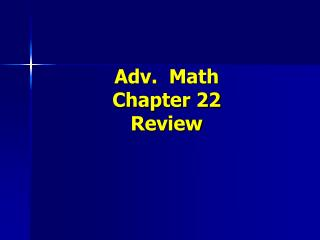DownloadDownload Presentation# Adv. Math Chapter 22 Review

Download Presentation## Adv. Math Chapter 22 Review

- - - - - - - - - - - - - - - - - - - - - - - - - - - E N D - - - - - - - - - - - - - - - - - - - - - - - - - - -
##### Presentation Transcript

2. Use the picture to write the ratios. Tell whether the ratio compares part to part, part to whole, or whole to part. All shapes to triangles. Rectangles to ovals. Ovals to all shapes.

3. Use the picture to write the ratios. Tell whether the ratio compares part to part, part to whole, or whole to part. All shapes to triangles. 18 : 9 whole to part Rectangles to ovals. 3 : 6 part to part Ovals to all shapes. 6 : 18 part to whole

4. Which of the following shows twoequivalent ratios? • a. 7 : 9 and 14 : 16 • 7 : 9 and 14 : 18

5. Which of the following shows twoequivalent ratios? • 7 : 9 and 14 : 18 • 7 = 14 • 9 18

6. Write twoequivalent ratios for each of the following. a. 12 : 15 b. 1 3

7. Write twoequivalent ratios for each of the following. a. 12 : 15 24 : 304 : 5 b. 123 3 6 9 *Note: There is more than 1 right answer.

8. Tell whether the ratios form a proportion. Write yes or no. 4 and2624and27 10 65 6 9

9. Tell whether the ratios form a proportion. Write yes or no. 4 and2624and27 10 65 6 9 Yes No

10. Solve the following proportions using Cross Products. Show your work!! 8 = x9 = 12 36 54 x 20

11. Solve the following proportions using Cross Products. Show your work!! 8 = x9 = 12 36 54 x 20 36x = 8(54) 12x = 9(20) 36x = 43212x = 180 36 36 12 12 x = 12 x = 15

12. Use the formula Distance = Rate x Time(d = rt)to solve the following:Valerie runs 1,200 meters in 8 minutes. What is her rate per minute?

13. Valerie runs 1,200 meters in 8 minutes. What is her rate per minute?d = r t1,200 = 8r 8 = 8150 meters per minute = rate

14. Use the formula Distance = Rate x Time(d = rt)to solve the following:Mr. Smith drove 275 miles at a rate of 50 miles per hour. How long did it take Mr. Smith to complete his trip?

15. Mr. Smith drove 275 miles at a rate of 50 miles per hour. How long did it take Mr. Smith to complete his trip?d = r t275 = 50 t 50 = 505.5 hours = time

16. Are the figures similar?

17. Are the figures similar? 6 = 10 3 5 30 = 30 YES

18. Are the figures similar? 4 = 2 2 1 4 = 4 YES

19. Are the figures similar?*Hint: Pay attention to position! 6 = 14.4 10 24 144 = 144 YES

20. What is the unit rate ? Show your work!! a. Earn \$56 for an 8 hour day b. Score 120 points in 15 games

21. What is the unit rate ? Show your work!! • \$\$\$56 = x • hours 8 1 x = \$7 per hour • b. points120 = x • games 15 1 x = 8 points per game

22. If the map scale is 1 in. = 15 miles, what is the map distance if the actual distance is 60 miles?

23. If the map scale is 1 in. = 15 miles, what is the map distance if the actual distance is 60 miles? Inch 1 = xMiles 15 6015x = 1(60)15x = 6015 15x = 4 inches

24. It takes Kenny 25 minutes to inflate the tires of 50 bicycles. How long will it take him to inflate the tires of 120 bicycles?

25. It takes Kenny 25 minutes to inflate the tires of 50 bicycles. How long will it take him to inflate the tires of 120 bicycles?minutes 25 = x bicycles 50 120 50x = 25 (120)50x = 3,000 50 50x = 60 minutes

26. How many pizzas do you need for a party of 135 people if at the last party, 90 people ate 52 pizzas?

27. How many pizzas do you need for a party of 135 people if at the last party, 90 people ate 52 pizzas?pizzas 52 = x people 90 135 90x = 52 (135)90x = 7,020 90 90x = 78 pizzas

28. Practice, Practice, Practice!